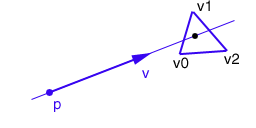Prev: Ray-Sphere Intersection Next: Catmull-Rom Spline

Given a ray, i.e. a parametric line equation, and a triangle, do they intersect? and if so what is the intersection point?

The solution presented in here is the one from Moller and Trumbore. A point in a triangle can be defined as:

```    	point(u,v) = (1-u-v)*p0 + u*p1 + v*p2

where

p0,p1,p2 are the vertices of the triangle

u >= 0
v >= 0
u + v <= 1.0```

We also know that the parametric equation of the line is:

```    	point(t) = p + t * d

where

p is a point in the line
d is a vector that provides the line's direction```

So if there is a point that belongs both to the line and the triangle we get:

`	p + t * d = (1-u-v) * p0 + u * p1 + v * p2`Therefore the intersection problem can be redefined as: is there a triplet (t,u,v) that satisfies the equation above, and complies with the restrictions for u and v? If the answer is yes, then the ray intersects the triangle, otherwise it doesn’t. For the maths details of solving this problem see either the reference above or check out the book “Real Time Rendering”. The following C code can be used to test the intersection:

```

/* a = b - c */
#define vector(a,b,c) \
(a) = (b) - (c);	\
(a) = (b) - (c);	\
(a) = (b) - (c);

int rayIntersectsTriangle(float *p, float *d,
float *v0, float *v1, float *v2) {

float e1,e2,h,s,q;
float a,f,u,v;
vector(e1,v1,v0);
vector(e2,v2,v0);

crossProduct(h,d,e2);
a = innerProduct(e1,h);

if (a > -0.00001 && a < 0.00001)
return(false);

f = 1/a;
vector(s,p,v0);
u = f * (innerProduct(s,h));

if (u < 0.0 || u > 1.0)
return(false);

crossProduct(q,s,e1);
v = f * innerProduct(d,q);

if (v < 0.0 || u + v > 1.0)
return(false);

// at this stage we can compute t to find out where
// the intersection point is on the line
t = f * innerProduct(e2,q);

if (t > 0.00001) // ray intersection
return(true);

else // this means that there is a line intersection
// but not a ray intersection
return (false);

`}````

Check out the cross product and the inner product definitions if you need help.

The code above only tells you if the ray intersects or not the triangle. If you want to know where then you can easily alter the code to return the triplet (t,u,v). Using the return value of t, or u and v, the intersection point, i.e. the values x,y,z where the ray intersects the triangle, can be found.

 Prev: Ray-Sphere Intersection Next: Catmull-Rom Spline

### 9 Responses to “Ray-Triangle Intersection”

1.You should reference http://paulbourke.net/

Thanks for pointing out t is f * innerProduct though. Now I can sort my hits by depth.

2.Hi! I am trying to determine multiple collision points and deformation of elastic objects. Will a single ray be sufficient to check multiple triangles (i.e. triangle mesh) intersection?

3.Ok it seems the (t, u, v) is indeed the intersection relative to the ray’s starting point (p). Am I correct?

•Hi,

The intersection point is pi = p + t*d; The variable t, computed in the routine, indicates the length we will have to follow direction d, from point p to intersect the triangle.

Hope this helps,

Antonio

•That helps a lot ! Exactly what I needed! Thank you !!

4.So is (t, u, v) the (x, y, z) (respectively) intersection ? If not how do I get the (x, y, z) intersection ?

•‘ u ‘ & ‘ v ‘ are the barycentric (not cartesian) co-ordinates of the intersection point.

‘ t ‘ is the parameter you use to define your ray equation:

intersection_point = position + (t * direction);

You know your position and direction vectors already, ‘ p ‘ and ‘ d ‘, so re-write the code above to tell you what ‘ t ‘ is. Then plug that value of ‘ t ‘ into the ray equation I wrote above, and hey presto, you have your intersection point.

5.Hi can you please tell me how to determine the x, y, z because I really don’t know how to for eg the values of u and v to determine it…

•To get (x, y, z):

if (t > 0.00001){

float finalPoint;

finalPoint = p + d * t;
finalPoint = p + d * t;
finalPoint = p + d * t;

return(true);
}else
return (false);

This site uses Akismet to reduce spam. Learn how your comment data is processed.